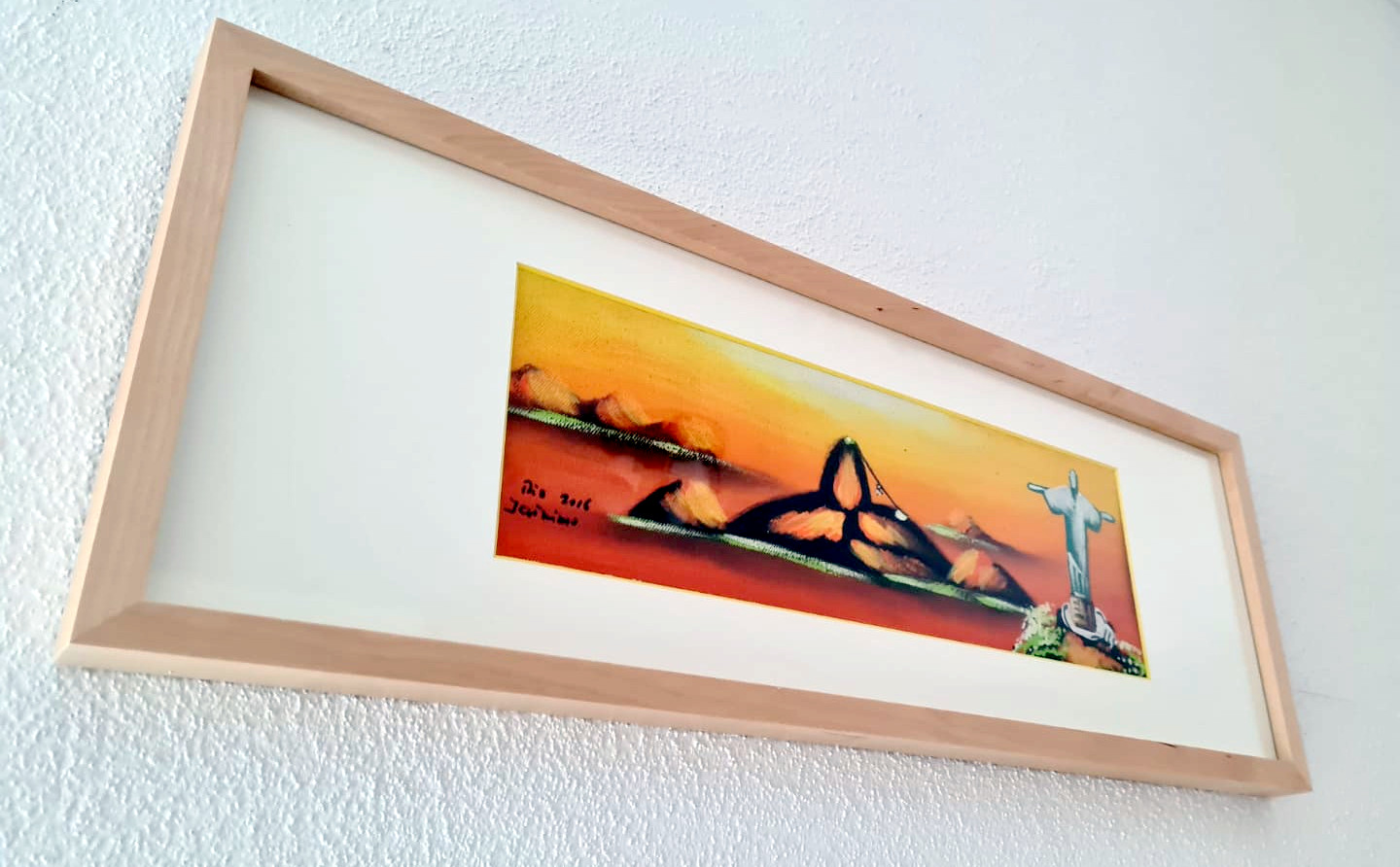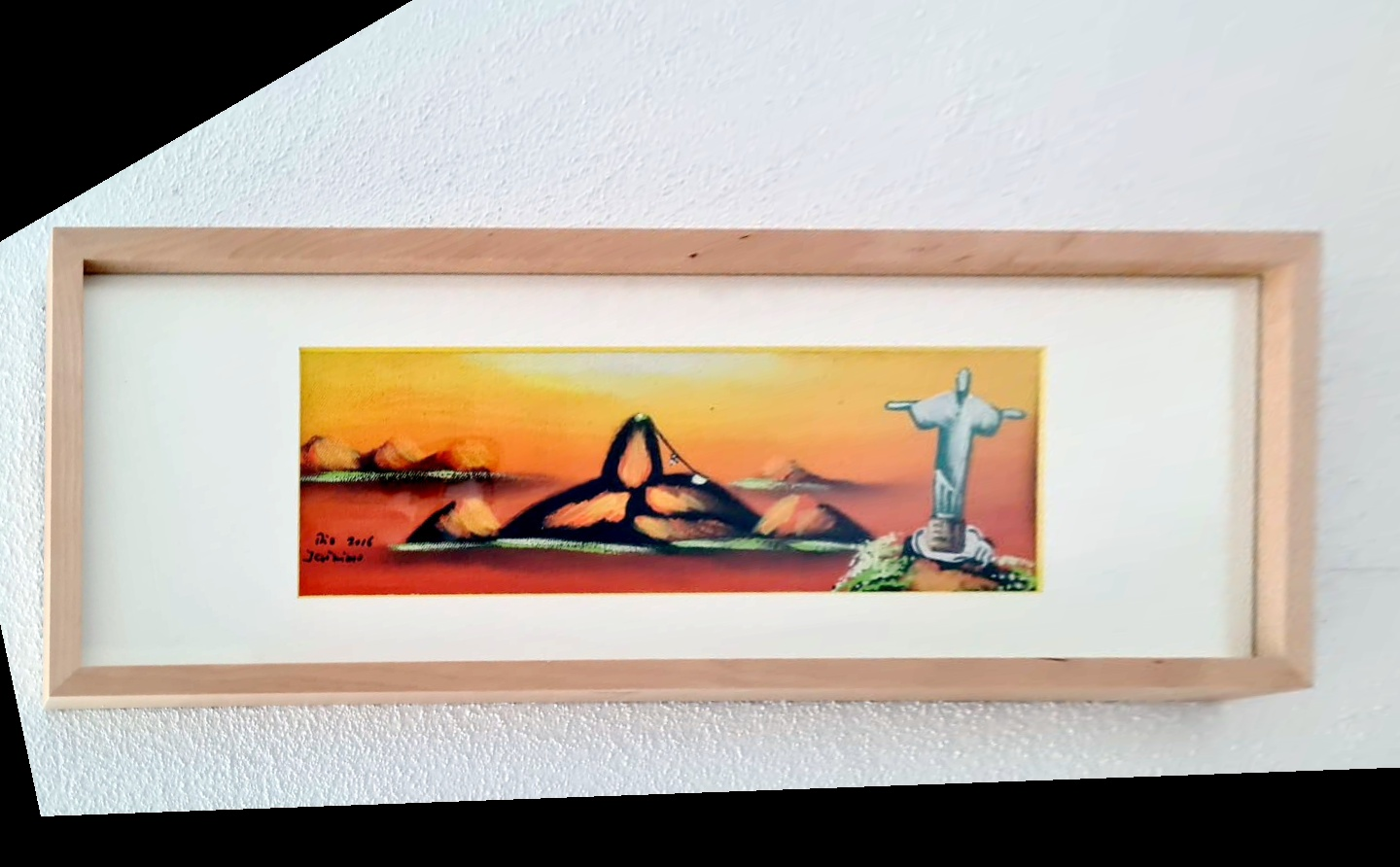Perspective Warp

# Overview

Perspective Warp algorithm allows for correcting perspective distortion caused by camera misalignment with respect to the object plane being captured. This is the case when the camera is, for instance, pointing to a frame hanging on a wall, but looking from below. The resulting image won't have opposite sides that are parallel.

If the camera position, tilt and pan relative to the frame are known, a 3x3 pespective transform can be derived, which will warp the image in order to keep frame's opposite sides parallel to each other, as shown below.

Input Transform Corrected\begin{bmatrix} 0.5386 & 0.1419 & -74\\ -0.4399 & 0.8662 & 291.5\\ -0.0005 & 0.0003 & 1 \end{bmatrix}# Implementation

The perspective transform matrix maps the source image into the destination image. The transform can be described mathematically by the equation below:

\begin{align*} \mathsf{y} = \mathsf{H}_p \mathsf{x} = \begin{bmatrix} \mathsf{A} & \mathsf{t} \\ \mathsf{p}^\intercal & p \end{bmatrix} \mathsf{x} \end{align*}

or, expanding the matrices and vectors:

\begin{align*} \begin{bmatrix} y_u \\ y_v \\ y_w \end{bmatrix} &= \begin{bmatrix} a_{11} & a_{12} & t_u \\ a_{21} & a_{22} & t_v \\ p_0 & p_1 & p \end{bmatrix} \begin{bmatrix}x_u \\ x_v \\ 1 \end{bmatrix} \\ \end{align*}

In these equations,

• $$\mathsf{H}_p$$ is the projection matrix
• $$\mathsf{x}$$ is the homogeneous coordinates in the source image.
• $$\mathsf{y}$$ is the homogeneous coordinates in the destination image.
• $$\mathsf{A}$$ is a 2x2 non-singular matrix with the linear component.
• $$\mathsf{t}$$ is the translation component.
• $$\mathsf{p},p$$ are the projective components. $$p$$ is usually 1.

The projection of $$\mathsf{y}$$ onto the output image is then given by:

\begin{align*} \begin{bmatrix} y'_u \\ y'_v \end{bmatrix} &= \begin{bmatrix} y_u/y_w \\ y_v/y_w \end{bmatrix} \end{align*}

These equations are efficiently implemented by doing the reverse operation, i.e, applying the inverse transform on destination pixels and sample the corresponding values from source image. If flag VPI_WARP_INVERSE is passed, the operation will assume that user's matrix is already inverted and won't try to invert it again. Pass zero if matrix must be inverted by VPI.

\begin{align*} \mathsf{H}_p^{-1} &= \begin{bmatrix}h_{11} & h_{12} & h_{13} \\ h_{21} & h_{22} & h_{23} \\ h_{31} & h_{32} & h_{33}\end{bmatrix} \\ \mathrm{dst}(u,v) &= \mathrm{src}\left(\frac{h_{11}u+h_{12}v+h_{13}}{h_{31}u+h_{32}v+h_{33}},\frac{h_{21}u+h_{22}v+h_{23}}{h_{31}u+h_{32}v+h_{33}}\right), \forall (u,v) \in \mathrm{dst} \end{align*}

# C API functions

For list of limitations, constraints and backends that implements the algorithm, consult reference documentation of the following functions:

Function Description
vpiSubmitPerspectiveWarp Submits a Perspective Warp operation to the stream.

# Usage

Language:
1. Import VPI module
import vpi
2. Define the 3x3 perspective transform to be applied.
xform = [[ 0.5386, 0.1419, -74 ],
[ -0.4399, 0.8662, 291.5 ],
[ -0.0005, 0.0003, 1 ]]
3. Applies the perspective transform in the VPI image input, returning the result in a new VPI image.
with vpi.Backend.CUDA:
output = input.perspwarp(xform)
1. Initialization phase
1. Include the header that defines the Perspective Warp function.
Declares functions that implement the Perspective Warp algorithm.
2. Define the input image object.
VPIImage input = /*...*/;
struct VPIImageImpl * VPIImage
A handle to an image.
Definition: Types.h:256
3. Create the output image. In this particular case both input and output have same dimensions, but they could be different. The image formats must match, though.
int32_t w, h;
vpiImageGetSize(input, &w, &h);
vpiImageGetFormat(input, &type);
VPIImage output;
vpiImageCreate(w, h, type, 0, &output);
uint64_t VPIImageFormat
Pre-defined image formats.
Definition: ImageFormat.h:94
VPIStatus vpiImageGetFormat(VPIImage img, VPIImageFormat *format)
Get the image format.
VPIStatus vpiImageCreate(int32_t width, int32_t height, VPIImageFormat fmt, uint64_t flags, VPIImage *img)
Create an empty image instance with the specified flags.
VPIStatus vpiImageGetSize(VPIImage img, int32_t *width, int32_t *height)
Get the image dimensions in pixels.
4. Create the stream where the algorithm will be submitted for execution.
VPIStream stream;
vpiStreamCreate(0, &stream);
struct VPIStreamImpl * VPIStream
A handle to a stream.
Definition: Types.h:250
VPIStatus vpiStreamCreate(uint64_t flags, VPIStream *stream)
Create a stream instance.
2. Processing phase
1. Define the 3x3 perspective transform to be applied. Note that the transform doesn't have to be the same in every call.
{
{ 0.5386, 0.1419, -74 },
{-0.4399, 0.8662, 291.5},
{-0.0005, 0.0003, 1 }
};
float VPIPerspectiveTransform
Represents a 2D perspective transform.
Definition: Types.h:590
2. Submit the algorithm to the stream, along with all parameters. The algorithm will be executed by the backend the CPU backend.
VPIStatus vpiSubmitPerspectiveWarp(VPIStream stream, uint64_t backend, VPIImage input, const VPIPerspectiveTransform xform, VPIImage output, const VPIWarpGrid *grid, VPIInterpolationType interp, VPIBorderExtension border, uint64_t flags)
Submits a Perspective Warp operation to the stream.
@ VPI_BACKEND_CPU
CPU backend.
Definition: Types.h:92
@ VPI_BORDER_ZERO
All pixels outside the image are considered to be zero.
Definition: Types.h:278
@ VPI_INTERP_LINEAR
Linear interpolation.
Definition: Interpolation.h:93
3. Optionally, wait until the processing is done.
vpiStreamSync(stream);
VPIStatus vpiStreamSync(VPIStream stream)
Blocks the calling thread until all submitted commands in this stream queue are done (queue is empty)...
3. Cleanup phase
1. Free resources held by the stream, the input and output images.
vpiImageDestroy(output);
void vpiImageDestroy(VPIImage img)
Destroy an image instance.
void vpiStreamDestroy(VPIStream stream)
Destroy a stream instance and deallocate all HW resources.

For more information, see Perspective Warp in the "C API Reference" section of VPI - Vision Programming Interface.

# Performance

For information on how to use the performance table below, see Algorithm Performance Tables.
Before comparing measurements, consult Comparing Algorithm Elapsed Times.
For further information on how performance was benchmarked, see Performance Benchmark.

-